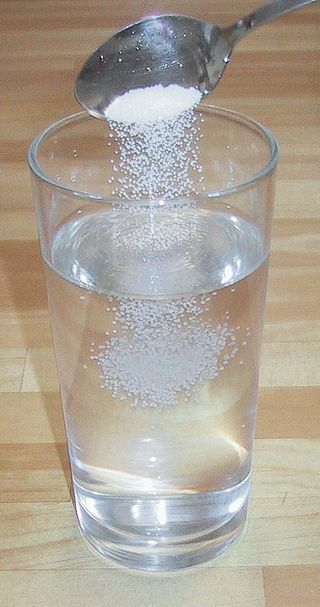# 9.4: Solutions - Homogeneous Mixtures

•• Anonymous
• LibreTexts
$$\newcommand{\vecs}{\overset { \rightharpoonup} {\mathbf{#1}} }$$ $$\newcommand{\vecd}{\overset{-\!-\!\rightharpoonup}{\vphantom{a}\smash {#1}}}$$$$\newcommand{\id}{\mathrm{id}}$$ $$\newcommand{\Span}{\mathrm{span}}$$ $$\newcommand{\kernel}{\mathrm{null}\,}$$ $$\newcommand{\range}{\mathrm{range}\,}$$ $$\newcommand{\RealPart}{\mathrm{Re}}$$ $$\newcommand{\ImaginaryPart}{\mathrm{Im}}$$ $$\newcommand{\Argument}{\mathrm{Arg}}$$ $$\newcommand{\norm}{\| #1 \|}$$ $$\newcommand{\inner}{\langle #1, #2 \rangle}$$ $$\newcommand{\Span}{\mathrm{span}}$$ $$\newcommand{\id}{\mathrm{id}}$$ $$\newcommand{\Span}{\mathrm{span}}$$ $$\newcommand{\kernel}{\mathrm{null}\,}$$ $$\newcommand{\range}{\mathrm{range}\,}$$ $$\newcommand{\RealPart}{\mathrm{Re}}$$ $$\newcommand{\ImaginaryPart}{\mathrm{Im}}$$ $$\newcommand{\Argument}{\mathrm{Arg}}$$ $$\newcommand{\norm}{\| #1 \|}$$ $$\newcommand{\inner}{\langle #1, #2 \rangle}$$ $$\newcommand{\Span}{\mathrm{span}}$$$$\newcommand{\AA}{\unicode[.8,0]{x212B}}$$

##### Learning Objectives
• Learn terminology involving solutions.

Attractive forces are also important for the formation of solutions. We will discuss why attractive forces are important in one of the subsections of this chapter, but first we will define a few terms that are important for solutions.

The major component of a solution is called the solvent. The minor component of a solution is called the solute. By major and minor we mean whichever component has the greater or lesser presence by mass or by moles. Sometimes this becomes confusing, especially with substances with very different molar masses. However, here we will confine the discussion to solutions for which the major component and the minor component are obvious.

Solutions exist for every possible phase of the solute and the solvent. Salt water, for example, is a solution of solid $$\ce{NaCl}$$ in liquid water, while air is a solution of a gaseous solute (O2) in a gaseous solvent (N2). In all cases, however, the overall phase of the solution is the same phase as the solvent. Table $$\PageIndex{1}$$ lists some common types of solutions, with examples of each.

Table $$\PageIndex{1}$$: Types of Solutions
Solvent Phase Solute Phase Example
gas gas air
liquid gas carbonated beverages
liquid liquid ethanol (C2H5OH) in H2O (alcoholic beverages)
liquid solid salt water
solid gas H2 gas absorbed by Pd metal
solid liquid Hg(ℓ) in dental fillings
solid solid steel alloysFigure $$\PageIndex{1}$$: Making a saline water solution by dissolving table salt (NaCl) in water. The salt is the solute and the water the solvent. (CC-BY-SA 3.0; Chris 73).
##### Example $$\PageIndex{1}$$: Sugar and Water

A solution is made by dissolving 1.00 g of sucrose ($$\ce{C12H22O11}$$) in 100.0 g of liquid water. Identify the solvent and solute in the resulting solution.

Solution

Either by mass or by moles, the obvious minor component is sucrose, so it is the solute. Water—the majority component—is the solvent. The fact that the resulting solution is the same phase as water also suggests that water is the solvent.

##### Exercise $$\PageIndex{1}$$

A solution is made by dissolving 3.33 g of $$\ce{HCl(g)}$$ in 40.0 g of liquid methyl alcohol ($$\ce{CH3OH}$$). Identify the solvent and solute in the resulting solution.

solute: HCl(g); solvent: CH3OH

## Summary

• Solutions are composed of a solvent (major component) and a solute (minor component).